Previous Year Questions Answers: Atoms and Molecules

# Previous Year Questions Answers: Atoms and Molecules - Science Class 9

Q.1. (a) Write the chemical formula of magnesium chloride and sodium hydroxide.
(b) Calculate the molecular mass of nitric acid (HNO3).    [H = 1 u, N = 14 u, O = 16 u]     [NCT 2019]
Ans.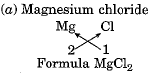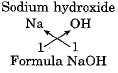(b) Molecular mass of nitric acid HNO3
= (1 x 1 u) + (1 x 14 u) + (3 x 16 u)
= 1 u + 14 u + 48 u

= 63 u

Q.2. Find which would weigh more 2 mole of CaCO3 or 10 mole of H2O.    [NCT 2019]
Ans.
1 mole of CaC03 weighs = (1 x 40 g) + (1 x 12 g) + (3 x 16 g)

= 40 + 12 + 48 = 100 g
2 moles of CaCO3 weigh = 2 x 100 g = 200 g
1 mole of H2O weighs = (2 x 1 g) + (1 x 16 g)
= 2 g + 16 g = 18 g
10 moles of H2O weigh = 10 x 18 g = 180 g
Therefore, 2 mole of CaCO3 weigh more.

Q.3. (i) Write the chemical formula of sodium oxide.
(ii) Calculate formula unit mass of ZnO. [Zn = 65, O = 16]
(iii) Define ion.    [NCT 2018]
Ans
. (i) Na2O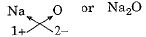(ii) Formula unit mass of ZnO = (65 u + 16 u) = 81 u
(iii) An atom or group of atoms carrying positive or negative charge is called an ion. For example, CI-, chloride ion.

Q.4. Find the number of moles in:    [NCT 2018]
(i) 176 g carbon dioxide.
(ii) 12.044 x 1023 number of carbon dioxide molecules.
(iii) Oxygen atoms in 44 g of carbon dioxide. [Atomic mass: C = 12 u, O = 16 u]
Ans.
(i) Molecular mass of CO2 =  12 + 32 = 44 u
44 g of CO2 = 1 mole
176 g of CO2 =(ii) 6.022 x 1023 molecules of CO2 = 1 mole
12.044 x 1023 molecules of CO2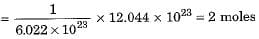(iii) 44 g CO2 means 1 mole of CO2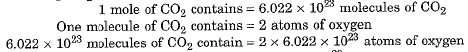= 12.044 x 1023 atoms of oxygen

Q.5. State any four postulates of Dalton’s atomic theory of matter. Which of his postulates does not hold correct at present?    [CBSE 2016]
Ans.
The postulates of Dalton’s atomic theory of matter are:
(i) All matter is made of very tiny particles called an atom.
(ii) Atoms of a given element are identical in mass and chemical properties.
(iii) Atoms of different elements have different masses and chemical properties.
(iv) Atoms combine in the ratio of simple whole numbers to form compounds.
(v) Atoms are indivisible particles that cannot be created or destroyed in a chemical reaction.
The postulate that atoms are indivisible particles does not hold good at present. We know that an atom is made up of protons, neutrons and electrons.

Q.6. (a) Define atomicity.
(b) State the atomicity of the following molecules:
(i) Oxygen
(ii) Phosphorous
(iii) Sulphur
(iv) Argon    [CBSE 2016]

Ans. (a) The number of atoms in one molecule of the substance is called atomicity.
(b) Atomicity of the substances is given as under: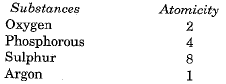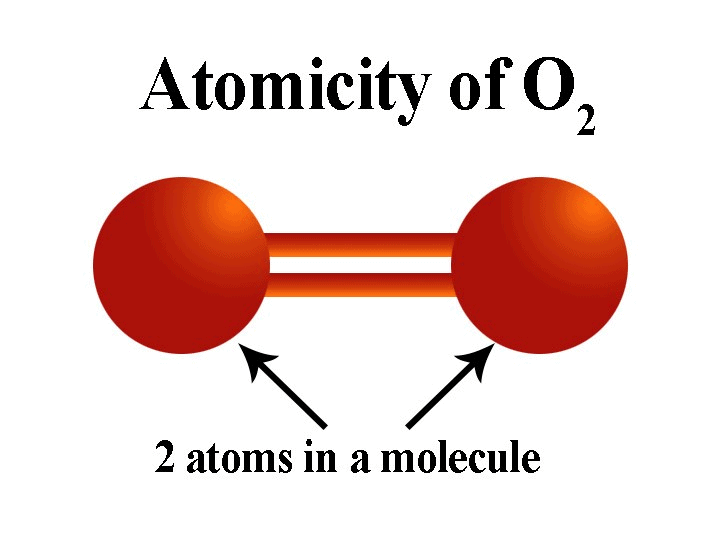Q.7. What is Avogadro’s constant?    [CBSE 2016]
Ans.
Avogadro’s constant is the number of particles (atoms, ions or molecules) present in one mole of the substance. Its value is 6.022 x 1023.

Q.8. The atomic number of three elements A, B and C are 9, 10 and 13 respectively. Which of them will form a cation?    [CBSE 2016]
Ans.
The element with the atomic number 13 will form a cation. It has electronic configuration 2, 8, 3. It loses three electrons to attain a stable octet and forms the cation.

Q.9. Name the isotope of an element that is used in the treatment of cancer.    [CBSE 2016]
Ans.
An isotope of cobalt is used in the treatment of cancer.

Q.10. The atomic mass of Calcium is 40 u. What will be the number of Calcium atoms in 0.4 u of Calcium?    [CBSE 2016]
Ans. 40 u of calcium contains 1 atom
0.4 u of calcium contains 1/40 x 0.4 = 10-2 atoms

Q.11. (i) Write the full form of IUPAC.
(ii) Hydrogen and oxygen combine in the ratio of 1: 8 by mass to form water. What mass of oxygen gas would be required to react completely with 3 g of hydrogen gas?    [CBSE 2016]
Ans.
(i) International Union of Pure and Applied Chemistry
(ii) 1 g of Hcombines with 8 g of oxygen. 3 g of H2 combines with 8 x 3 = 24 g of oxygen.

Q.12. Name the international organisation which approves the name given to the elements.    [CBSE 2016]
Ans.
IUPAC, the International Union of Pure and Applied Chemistry.

Q.13. As per the law of definite proportions carbon and oxygen combine in a ratio of 3:8. Compute the mass of oxygen gas that would be required to react completely with 6 g carbon.     [CBSE 2016]
Ans.
3 g of carbon combines with 8 g of oxygen
∴ 6 g of carbon will combine with = 8/3 x 6 =16 g of oxygen.

Q.14. (a) Which among the following has more number of molecules:
1 g of hydrogen (H2) or 1 g of methane (CH4)?
(Atomic mass of H = 1 u, C = 12 u)
(b) Calculate the number of particles in 46 g of Na atoms.
(Atomic mass of Na = 23 u)    [CBSE 2016]
Ans.
(a) 1 g of H2 contains number of molecules
= 1/2 x 6.022 x 1023
= 3.011 x 1023 molecules
1 g of CH4 contains number of molecules
= 1/16 x 6.022 x 1023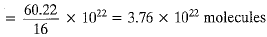∴ 1 g of H2 contains more number of molecules.
(b) The atomic mass of Na atom = 23 g
∵ 23 g Na atom contains 6.022 x 1023 particles
∴ 46 g Na atom will contain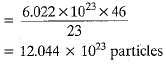Q.15. The oxide of aluminium has a chemical formula AI2O3. State the valency of AL.    [CBSE 2015]
Ans.
The valency of AI = 3.

Q.16. State three points of difference between anion and cation.    [CBSE 2015]
Ans.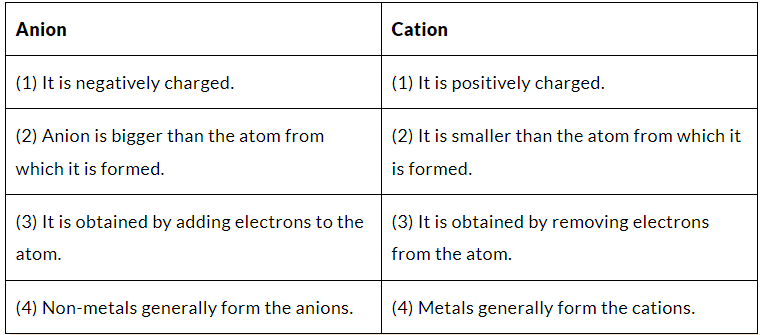Q.17. What is AMU? Which quantity is measured in terms of AMU?    [CBSE 2015]
Ans.
AMU stands for Atomic Mass Unit.
Atomic mass is measured in terms of AMU.

Q.18. Who gave the laws of chemical combination?    [CBSE 2015]
Ans.
Lavoisier and Proust

Q.19. What do you understand by an octet of electrons in the valence shell?    [CBSE 2015]
Ans.
If there are eight electrons in the outermost shell of an atom, we say that it has an octet of electrons. All atoms have a tendency to acquire octet of electrons in the valence shell.

Q.20. (a) Define one mole. How is it related to Avogadro’s constant?
(b) Find the number of sodium ion in one mole of sodium sulphate.    [CBSE 2015]
Ans.
(a) 1 mole is defined as equal to 6.022 x 1023 particles. It is also equal to molar mass in grams.
= 6.022 x 1023
(b) 1 mole of Na2SO4 contains 2 moles of Na+
2 moles of Na+ = 2 x 6.022 x 1023
= 12.044 x 1023 = 1.2044 x 1024Na+ ions.

Q.21. Define atomicity. Give an example of each of monoatomic, diatomic, tetra-atomic and polyatomic molecules.    [CBSE 2015]
Ans. Atomicity is defined as the number of atoms present in a molecule. He is monoatomic, H2 is diatomic, P4 is tetra-atomic and S8 is polyatomic molecules.

Q.1. (a) What is meant by molecular formula? State with example what information can be derived from a molecular formula?
(b) Write the names of the compounds represented by the following formulae:

(i) Mg(NO3)2
(ii) K2SO4
(iii) Ca3N2    [CBSE 2016]
Ans.
(a) Molecular formula of a compound shows the constituent elements and the number of atoms of each element present in one molecule of the compound.
Take the example of H2SO4. It is the molecular formula of sulphuric acid. It shows that
(i) Sulphuric acid molecule is constituted of hydrogen, sulphur and oxygen.
(ii) One molecule of sulphuric acid contains two atoms of hydrogen, one atom of sulphur and four atoms of oxygen.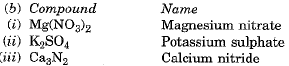Q.2. (i) Write chemical formulae of all the compounds that can be formed by the combination of the following ions: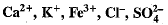(b) The molar mass of nitrogen is 14u. What will be the mass of one atom of nitrogen in grams?    [CBSE 2016]
Ans. (a)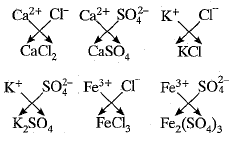(b) 1 mole of nitro g en atoms = 14 g
1 mole of nitrogen atoms
= 6.022 x 1023 atom
6.022 x 1023 atoms of nitrogen weigh = 14 g
1 atom of nitrogen weighs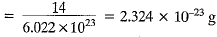Q.3. (a) Calculate the number of oxygen atoms in 0.10 mole of Na2CO3.10H2O.
(b) If one mole of sulphur weighs 32 grams, what is the mass (in grams) of 1 atom of sulphur?
(c) Identify the correct formula for ammonium sulphate from the following formula: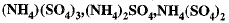[CBSE 2015]
Ans.
(a) 1 mole of Na2CO3.10H2O contains 13 x 6.022 x 1023 atoms of oxygen
∴ 0.10 moles of Na2CO3.10H2O will contain= 0.10 x 13 x 6.022 x 1023
= 7.83 x 1023 oxygen atoms.
(b) 1 mole of sulphur = 32 g
Also 1 mole of sulphur
= 6.022 x 1023 atoms
Now, 6.022 x 1023 atoms of sulphur weigh = 32g
∴ 1 atom of sulphur weighs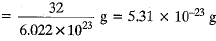(c) (NH4)2SO

The document Previous Year Questions Answers: Atoms and Molecules | Science Class 9 is a part of the Class 9 Course Science Class 9.
All you need of Class 9 at this link: Class 9

## Science Class 9

66 videos|355 docs|97 tests

## FAQs on Previous Year Questions Answers: Atoms and Molecules - Science Class 9

 1. What is an atom and molecule?Ans. An atom is the smallest unit of matter that retains the properties of an element. It consists of a positively charged nucleus surrounded by negatively charged electrons. A molecule, on the other hand, is a group of two or more atoms chemically bonded together. It can be of the same elements or different elements.
 2. How are atoms represented in chemical equations?Ans. Atoms are represented in chemical equations using their chemical symbols. Each element has a unique symbol, such as H for hydrogen, O for oxygen, and C for carbon. The number of atoms present is indicated by a subscript written after the chemical symbol. For example, H2O represents a molecule of water, consisting of two hydrogen atoms and one oxygen atom.
 3. How do atoms combine to form molecules?Ans. Atoms combine to form molecules through chemical bonding. There are two types of chemical bonding – covalent bonding and ionic bonding. In covalent bonding, atoms share electrons to complete their outermost electron shells. This results in the formation of molecules, such as H2 (hydrogen gas) or H2O (water). In ionic bonding, atoms transfer electrons to form ions, which then attract each other to form molecules, such as NaCl (sodium chloride) or CaCO3 (calcium carbonate).
 4. What is the difference between an element and a compound?Ans. An element is a pure substance made up of only one type of atom. It cannot be broken down into simpler substances by ordinary chemical means. Examples of elements are hydrogen, oxygen, and carbon. A compound, on the other hand, is a substance made up of two or more different elements chemically bonded together. It can be broken down into its constituent elements by chemical reactions. Examples of compounds are water (H2O) and carbon dioxide (CO2).
 5. How can you determine the number of atoms in a given molecule?Ans. To determine the number of atoms in a given molecule, you need to look at the chemical formula of the molecule. The numbers written as subscripts after the chemical symbols indicate the number of atoms present. For example, in the chemical formula H2O, there are two hydrogen atoms and one oxygen atom. In the chemical formula CO2, there is one carbon atom and two oxygen atoms. By counting the number of atoms based on the subscripts, you can determine the total number of atoms in the molecule.

## Science Class 9

66 videos|355 docs|97 testsExplore Courses for Class 9 examSignup to see your scores go up within 7 days! Learn & Practice with 1000+ FREE Notes, Videos & Tests.
10M+ students study on EduRev
Track your progress, build streaks, highlight & save important lessons and more!
Related Searches

,

,

,

,

,

,

,

,

,

,

,

,

,

,

,

,

,

,

,

,

,

;